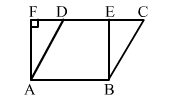# In the given figure, a || gm ABCD and a rectangle ABEF are of equal area. Then,

Question:

In the given figure, a || gm ABCD and a rectangle ABEF are of equal area. Then,

(a) perimeter of ABCD = perimeter of ABEF
(b) perimeter of ABCD < perimeter of ABEF
(c) perimeter of ABCD > perimeter of ABEF

(d) perimeter of $A B C D=\frac{1}{2}$ (perimeter of $A B E F$ )Solution:

(c) perimeter of ABCD > perimeter of ABEF

Parallelogram ABCD and rectangle ABEF lie on the same base AB, i.e., one side is common in both the figures.
In ||gm ABCD, we have: